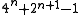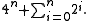xKynea numberEncyclopedia
A Kynea number is an integer of the form.

An equivalent formula is.

This indicates that a Kynea number is the nth power of 4 plus the (n + 1)th Mersenne number.
Kynea numbers were studied by Cletus Emmanuel who named them after a baby girl.

The sequence of Kynea numbers starts with:
7, 23
23 (number)
23 is the natural number following 22 and preceding 24.- In mathematics :Twenty-three is the ninth prime number, the smallest odd prime that is not a twin prime. Twenty-three is also the fifth factorial prime, the third Woodall prime...

, 79
79 (number)
Seventy-nine is the natural number following 78 and preceding 80.79 may represent:-In mathematics:*An odd number*The smallest number that can't be represented as a sum of fewer than 19 fourth powers*A strictly non-palindromic number...

, 287, 1087, 4223, 16639, 66047, 263167, 1050623, 4198399, 16785407, ... .

## Properties

The binary representation
Binary numeral system
The binary numeral system, or base-2 number system, represents numeric values using two symbols, 0 and 1. More specifically, the usual base-2 system is a positional notation with a radix of 2...

of the nth Kynea number is a single leading one, followed by n - 1 consecutive zeroes, followed by n + 1 consecutive ones, or to put it algebraically:So, for example, 23 is 10111 in binary, 79 is 1001111, etc. The difference between the nth Kynea number and the nth Carol number is the (n + 2)th power of two
Power of two
In mathematics, a power of two means a number of the form 2n where n is an integer, i.e. the result of exponentiation with as base the number two and as exponent the integer n....

.

## Prime Kynea numbers

Starting with 7, every third Kynea number is a multiple of 7. Thus, for a Kynea number to be a prime number
Prime number
A prime number is a natural number greater than 1 that has no positive divisors other than 1 and itself. A natural number greater than 1 that is not a prime number is called a composite number. For example 5 is prime, as only 1 and 5 divide it, whereas 6 is composite, since it has the divisors 2...

, its index n can not be of the form 3x + 1 for x > 0. The first few Kynea numbers that are also prime are 7, 23, 79, 1087, 66047, 263167, 16785407 .

As of 2006, the largest known prime Kynea number has index n = 281621 and approximately equals 5.5×10169552. It was found by Cletus Emmanuel in November 2005, using k-Sieve from Phil Carmody and OpenPFGW. This is the 46th Kynea prime.